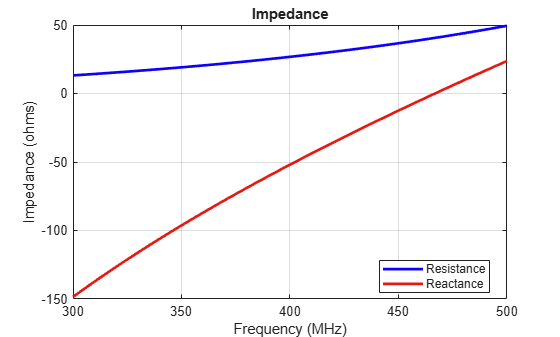# bowtieTriangular

Create planar bowtie dipole antenna

## Description

The `bowtieTriangular` object is a planar bowtie antenna on the yz- plane. The default planar bowtie dipole is center-fed. The feed point coincides with the origin. The origin is located on the yz- plane.## Creation

### Syntax

``bt = bowtieTriangular``
``bt = bowtieTriangular(Name,Value)``

### Description

``` `bt = bowtieTriangular` creates a half-wavelength planar bowtie antenna.```

example

``` `bt = bowtieTriangular(Name,Value)` creates a planar bowtie antenna with additional properties specified by one or more name-value pair arguments. `Name` is the property name and `Value` is the corresponding value. You can specify several name-value pair arguments in any order as `Name1`, `Value1`, `...`, `NameN`, `ValueN`. Properties not specified retain their default values.```

## Properties

expand all

Planar bowtie length, specified as a scalar in meters. By default, the length is chosen for the operating frequency of 410 MHz.

Example: `'Length',3`

Data Types: `double`

Planar bowtie flare angle near the feed, specified as a scalar in meters.

Note

Flare angle should be less than `175` degrees and greater than `5` degrees.

Example: `'FlareAngle',80`

Data Types: `double`

Type of the metal used as a conductor, specified as a metal material object. You can choose any metal from the `MetalCatalog` or specify a metal of your choice. For more information, see `metal`. For more information on metal conductor meshing, see Meshing.

Example: ```m = metal('Copper'); 'Conductor',m```

Example: ```m = metal('Copper'); ant.Conductor = m```

Lumped elements added to the antenna feed, specified as a lumped element object. For more information, see `lumpedElement`.

Example: `'Load'`, lumpedelement. `lumpedelement` is the object for the load created using `lumpedElement`.

Example: ```bt.Load = lumpedElement('Impedance',75)```

Tilt angle of the antenna, specified as a scalar or vector with each element unit in degrees. For more information, see Rotate Antennas and Arrays.

Example: `'Tilt',90`

Example: `ant.Tilt = 90`

Example: `'Tilt',[90 90]`,`'TiltAxis',[0 1 0;0 1 1]` tilts the antenna at 90 degrees about the two axes defined by the vectors.

Note

The `wireStack` antenna object only accepts the dot method to change its properties.

Data Types: `double`

Tilt axis of the antenna, specified as:

• Three-element vector of Cartesian coordinates in meters. In this case, each coordinate in the vector starts at the origin and lies along the specified points on the X-, Y-, and Z-axes.

• Two points in space, each specified as three-element vectors of Cartesian coordinates. In this case, the antenna rotates around the line joining the two points in space.

• A string input describing simple rotations around one of the principal axes, 'X', 'Y', or 'Z'.

Example: `'TiltAxis',[0 1 0]`

Example: `'TiltAxis',[0 0 0;0 1 0]`

Example: `ant.TiltAxis = 'Z'`

Note

The `wireStack` antenna object only accepts the dot method to change its properties.

Data Types: `double`

## Object Functions

 `show` Display antenna or array structure; display shape as filled patch `info` Display information about antenna or array `axialRatio` Axial ratio of antenna `beamwidth` Beamwidth of antenna `charge` Charge distribution on metal or dielectric antenna or array surface `current` Current distribution on metal or dielectric antenna or array surface `design` Design prototype antenna or arrays for resonance around specified frequency `efficiency` Radiation efficiency of antenna `EHfields` Electric and magnetic fields of antennas; Embedded electric and magnetic fields of antenna element in arrays `impedance` Input impedance of antenna; scan impedance of array `mesh` Mesh properties of metal or dielectric antenna or array structure `meshconfig` Change mesh mode of antenna structure `optimize` Optimize antenna or array using SADEA optimizer `pattern` Radiation pattern and phase of antenna or array; Embedded pattern of antenna element in array `patternAzimuth` Azimuth pattern of antenna or array `patternElevation` Elevation pattern of antenna or array `rcs` Calculate and plot radar cross section (RCS) of platform, antenna, or array `returnLoss` Return loss of antenna; scan return loss of array `sparameters` Calculate S-parameter for antenna and antenna array objects `vswr` Voltage standing wave ratio of antenna

## Examples

collapse all

Create and view a center-fed planar bowtie antenna that has a 60 degrees flare angle.

`b = bowtieTriangular('FlareAngle',60)`
```b = bowtieTriangular with properties: Length: 0.2000 FlareAngle: 60 Conductor: [1x1 metal] Tilt: 0 TiltAxis: [1 0 0] Load: [1x1 lumpedElement] ```
`show(b)`Calculate and plot the impedance of a planar bowtie antenna over a frequency range of 300 MHz-500 MHz.

```b = bowtieTriangular('FlareAngle',60); impedance(b,linspace(300e6,500e6,51))```Balanis, C.A.Antenna Theory: Analysis and Design.3rd Ed. New York: Wiley, 2005.

 Brown, G.H., and O.M. Woodward Jr. “Experimentally Determined Radiation Characteristics of Conical and Triangular Antennas”. RCA Review. Vol.13, No.4, Dec.1952, pp. 425–452